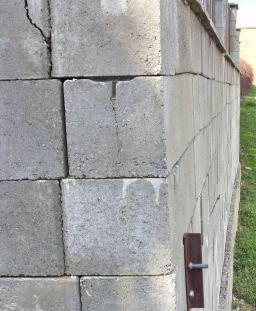# Cross-section 5089

The brick pillar with a cross-section of 41x77 cm has a height of 3.25 m. Calculate:

(a) the volume of the pillar
b) the required number of bricks (if we need 400 pieces per 1 cubic meter)

V =  1.026 m3
n =  411

### Step-by-step explanation:Did you find an error or inaccuracy? Feel free to write us. Thank you!

Tips for related online calculators
Do you want to convert length units?
Do you know the volume and unit volume, and want to convert volume units?
Do you want to round the number?

#### Grade of the word problem:

We encourage you to watch this tutorial video on this math problem: